# Designing a Car Suspension System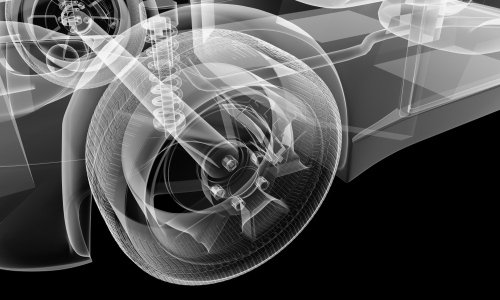## Determining the physical setup

A car suspension system should provide a comfortable ride when driving on a road with bumps, and should there be unwanted disturbance, we will want the system to quickly settle. To simplify the car suspension diagram, we will model 2 masses and 2 sets of springs and dampers Fig. 1a and analyze ¼ of an entire car.

\begin{array} {|l|l|}\hline \text{Car Mass} & 2500\text{ kg} \\ \hline \text{Body Mass (m1)} & 650\text{ kg} \\ \hline \text{Wheel and suspension mass (m2)}  & 150\text{ kg} \\ \hline  \end{array}

## Modeling the car suspension system

We will start by introducing forces into our system based on its elements.

The force from the spring can be shown as S ̅ which is proportional to displacement – the bigger the deformation the bigger the force. The value of the vector S ̅ can be represented using the following equation:

$$S = k∆$$

where $$k$$ is the spring constant and $$Δ$$ is the deformation of the spring

The force from the damper can be shown as G ̅ which is proportional to the speed of the deformation and can be represented using the following equation:

$$G=cv$$

where $$c$$ is the damping constant and $$v$$ the velocity of the mass

To represent the motion in the system, we will assume that F ̅ represents the forces acting on the system, x1 and x2 represent the displacement of the masses m1 and m2 respectively and w is the disturbance (e.g., a bump on the road)

### Motion equations:

$$m_1\ddot x_1=−S_1−G_1+F$$

$$m_2 \ddot x_2 = S_1' + G_1' -S_2 - G_2 - F$$

### Spring equations:

$$S_1=k_1\left(x_1-x_2\right)$$

$$S_2=k_2(x_2-w)$$

### Damper equations:

$$G_1 = c_1 (\dot x _1 - \dot x_2)$$

$$G_2 = c_2 (\dot x_2- \dot w)$$

Now we will model the following spring-damper-mass system in Collimator

$$\ddot x_1 = \frac{1}{m_1( -k_1x_1 - c_1\dot x_1 + F )}$$

1. Sign in to Collimator, create a new project and open the Model Editor
2. Insert an adder block from the Block Library since all values inside the round brackets are being added together
3. Add a gain block to multiply our signal by $$\frac{1}{m1}$$ to get the acceleration of mass $$m1$$
4. Add two integral blocks to get the mass’s velocity and displacement
5. Multiply both sequentially by constants $$c­1$$ and $$k1$$ to get the values we need to connect to back to the adder Block
6. Finally, add the control force, $$F$$ in the form of step signal

In this simulation we use following parameters:

\begin{array} {|l|l|}\hline k1 & 600\text{ N/m} \\ \hline c1 & 140\text{ Ns2/m} \\ \hline m1 & 650\text{ kg} \\ \hline  \end{array}

The Visualizer shows the resulting behavior of displacement of mass, m1. This system stabilizes itself with time, but slowly and only to a certain degree. Having this spring for our car would result in long oscillations that will likely make people sick during travel. You can experiment with values of parameters to see how the spring-damper-mass system responds to different values. Here are a few quick examples:

\begin{array} {|l|l|}\hline k1 & 600\text{ N/m} \\ \hline c1 & 140\text{ Ns2/m} \\ \hline m1 & 1300\text{ kg} \\ \hline  \end{array}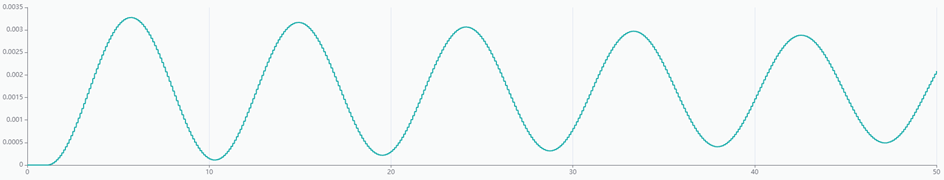Fig. 6a. Double the mass $$m1 = 1300 [kg]$$ causes longer oscillations

\begin{array} {|l|l|}\hline k1 & 600\text{ N/m} \\ \hline c1 & 140\text{ Ns2/m} \\ \hline m1 & 2600\text{ kg} \\ \hline  \end{array}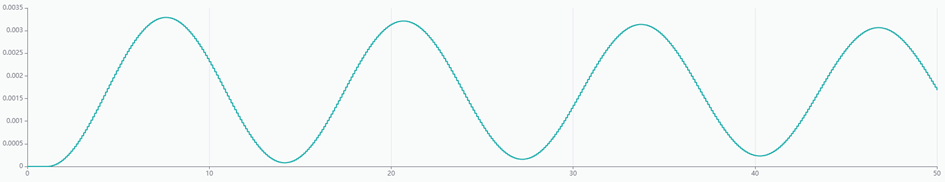Fig. 6b. 4x the mass $$m1 = 2600 [kg]$$ causes oscillations that are even longer

Increasing the spring coefficient $$k1 = 1200N/m$$ would result in losing stability. Decreasing the spring coefficient to$$k1 = 300 N/m$$ would make the system more stable, but at the cost of stronger oscillations at the beginning.

\begin{array} {|l|l|}\hline k1 & 1200\text{ N/m} \\ \hline c1 & 140\text{ Ns2/m} \\ \hline m1 & 650\text{ kg} \\ \hline  \end{array}

\begin{array} {|l|l|}\hline k1 & 300\text{ N/m} \\ \hline c1 & 140\text{ Ns2/m} \\ \hline m1 & 650\text{ kg} \\ \hline  \end{array}

Increasing the damping coefficient $$c1 = 280Ns2/m­­$$ would result in more stability and stronger start of the vibrations. Decreasing the damping coefficient $$c1 = 70 Ns2/m­­$$ would give the system stronger and more frequent oscillations.

\begin{array} {|l|l|}\hline k1 & 300\text{ N/m} \\ \hline c1 & 280\text{ Ns2/m} \\ \hline m1 & 650\text{ kg} \\ \hline  \end{array}

\begin{array} {|l|l|}\hline k1 & 300\text{ N/m} \\ \hline c1 & 70\text{ Ns2/m} \\ \hline m1 & 650\text{ kg} \\ \hline  \end{array}

To ensure the model is not unwieldy and hard to read, we will use the Submodel block to neatly package the suspension model we just built.

We can then expand our system to look at the effect on both masses m1 and m2 using the following equations:

$$\ddot x_1 = \frac{1}{m_1 ( -k_1 (x_1 - x_2) - c_1 (\dot x_1 - \dot x_2 ) + F )}$$

$$\ddot x_2 = \frac{1}{m_2 (k_1 (x_1 - x_2) + c_1 ( \dot x_1 - \dot x_2 ) - k_2 (x_2 - w) - c_2 ( \dot x_2 - \dot w) - F )}$$

To model the system in Collimator we will:

1. Insert a summation block from the block library for all elements in the parenthesis
2. Multiply the result by $$\frac{1}{m}$$ to get acceleration of both masses
3. Insert two integration blocks to get velocity and displacement for both masses

4. Add Gain blocks to multiply the displacement by the spring constant and velocity by the damping coefficient
5. Connect the corresponding blocks to complete the equation for first mass

6. Go through the same process to design the second second system

7. Finally, we will connect the diagram with two inputs (Inport blocks 0 (F) and 1 (w)) and one output (Outport block 0). The difference between displacement of both masses $$–x1-x2$$, is the output of this Submodel

We will then simulate the system using the following parameters and add a control force as a step signal at $$1s$$ with value of $$0.1N$$ and disturbance at $$10s$$ with value of $$0.1m$$ – simulating a bump on the road.

\begin{array} {|l|l|}\hline k1 & 600\text{ N/m} \\ \hline c1 & 140\text{ Ns2/m} \\ \hline m1 & 650\text{ kg} \\ \hline k2 & 620\text{ N/m} \\ \hline c2 & 380\text{ Ns2/m} \\ \hline m2 &150\text{ kg} \\ \hline F & 0.1\text{ N} \\ \hline w & 0.1\text{ m} \\ \hline  \end{array}

The following figures show response of our system with the control force and disturbance. It is visible in 10th second when the system starts to move erratically for a few seconds before calming down.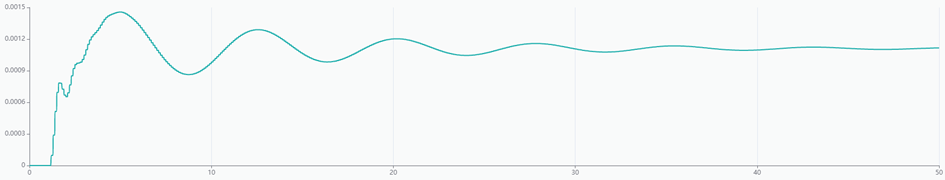Fig. 15a. Responses of suspension model: control force only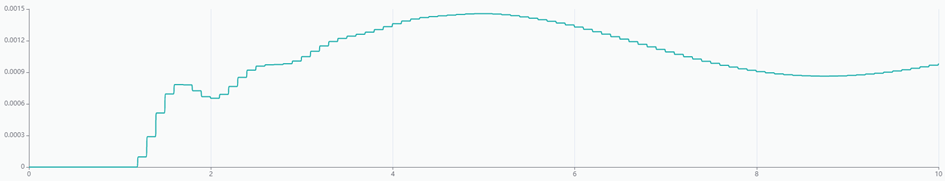Fig. 15b. Responses of suspension model: control force with disturbance – first 10 seconds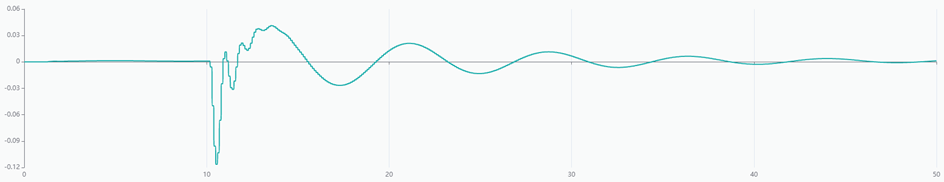Fig. 15c. Responses of suspension model: control force with disturbance - first 50 seconds

We can also analyze the system by looking at the displacement of both masses separately with just the control force. The first two figures show that mass m1 has at least 3 times more influence on the behavior of whole system than mass $$m2$$.

After adding disturbance, we see that it doesn’t affect the character of movement of mass m1 while mass $$m2$$ responds immediately. In addition, it would appear that the disturbance made the displacement 100 times bigger than in the previous case.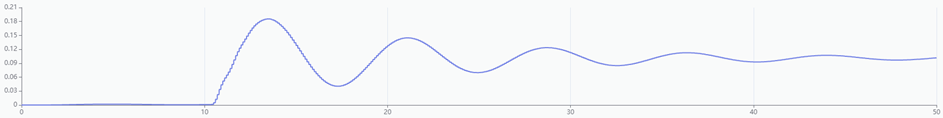Fig. 17a. Displacement of the first mass: control force with disturbance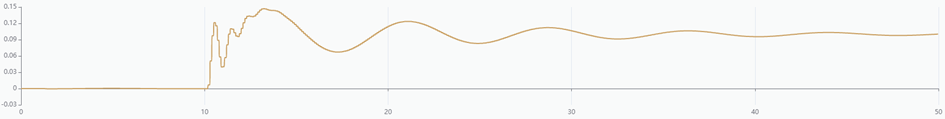Fig. 17b. Displacement of the second mass: control force with disturbance

From these charts we can already tell that the car ride would not be comfortable. To improve the experience for our driver and his passengers, we will introduce a controller.

## Designing the controller for the car suspension system

We will introduce a simple, yet versatile, feedback mechanism for our system: the Proportional-Integral-Derivative (PID) controller. The PID controller is widely used by engineers because it is easy to understand and takes into account the history of the system while also anticipating future behavior. The control function can be represented as follows:

$$u(t) = K_p + K_I \int_{0}^{t} e(\tau) d\tau + K_D * \frac{de(t)}{d}$$

where $$u(t)$$ is the control signal, $$e(t)$$ the error value, and $$KP$$, $$KI$$, $$KD$$ are coefficients for the proportional, integral, and derivative terms. Additionally, the error value will be computed as follows:

$$e(t) = r(t) - y(t)$$

where $$r(t)$$ is the desired setpoint of the process and $$y(t)$$ the process variable (in our case, the difference between displacements of the two masses).

Instead of using Collimator's PID controller block, we will create a new one using the following steps:

1. Drag and drop an adder to the Model Editor
2. Multiply the input by Kp
3. Compute the integral of the input and then multiply by the Ki coefficient
4. Compute the derivative of the input and then multiply by the Kd coefficient
5. Add all three signals together
6. Subtract the response of the system from signal to determine the value of error to feed back
We see the amplitude of the response signal $$(x1 – x2)$$ equals about $$0.01m or 1mm$$. Choosing PID parameters is whole different topic and will be handled in another tutorial. For this exercise we will manually tune the PID controller. The parameters that minimize the car suspension system oscillations seems to be close to: